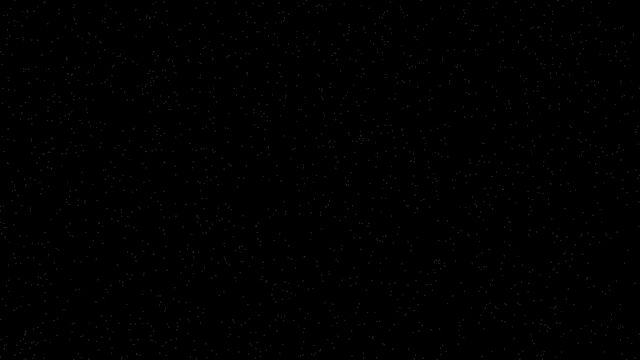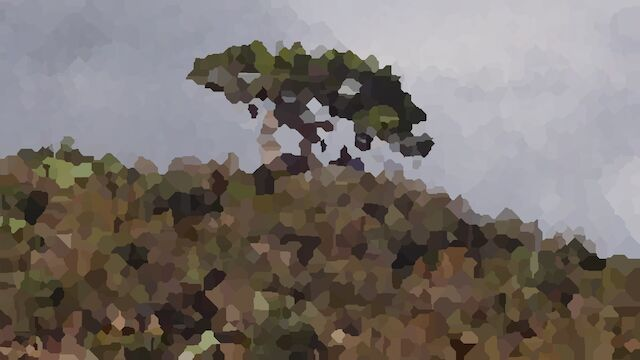# # Taxicab Voronoi

## # 1 Euclidean Metric

Distances are found by summing the square of the difference in each coordinate, then taking square root.

$d(p, q) = \sqrt{\sum (p_i - q_i)^2}$

## # 2 Taxicab Metric

Distances are found by summing the absolute difference in each coordinate.

$d(p, q) = \sum |p_i - q_i|$

## # 3 Voronoi Tesselation

Given a collection of marked points, assign a colour to each arbitrary point according to the colour of the nearest point.

Usually uses Euclidean metric.

## # 4 Taxicab Voronoi

Voronoi tesselation using taxicab metric can be implemented easily.

The idea is to do 4 passes, one in each direction of each coordinate. Keep track of the currently known nearest point for each pixel. Each pass can be parallized, with a sequential inner loop.

### # 4.1 Example

Image input:Control input:Output:### # 4.2 Reference Implementation

// taxicab-voronoi.c (C) 2023 Claude Heiland-Allen

#include <limits.h>
#include <stdio.h>
#include <stdlib.h>

// keep track of the nearest centre
struct pixel
{
int distance;
int x;
int y;
};

// voronoi pixelation in taxicab metric
// cell centres have a != 0
// pixels with a == 0 are set to rgb of nearest centre
int taxicab_voronoi(int width, int height, unsigned char *rgb, const unsigned char *a)
{
struct pixel *image = malloc(width * height * sizeof(*image));
if (! image)
{
return 1;
}
// initialize
#pragma omp parallel for collapse(2)
for (int y = 0; y < height; ++y)
{
for (int x = 0; x < width; ++x)
{
int k = y * width + x;
if (a[k])
{
image[k].distance = 0;
}
else
{
image[k].distance = INT_MAX;
}
image[k].x = x;
image[k].y = y;
}
}
// pass 1: increasing x
#pragma omp parallel for
for (int y = 0; y < height; ++y)
{
// sequential
for (int x = 1; x < width; ++x)
{
int k = y * width + x;
int k1 = y * width + (x - 1);
int d = image[k1].distance;
if (image[k].distance > d)
{
image[k].distance = d + 1;
image[k].x = image[k1].x;
image[k].y = image[k1].y;
}
}
}
// pass 2: decreasing x
#pragma omp parallel for
for (int y = 0; y < height; ++y)
{
// sequential
for (int x = width - 2; x >= 0; --x)
{
int k = y * width + x;
int k1 = y * width + (x + 1);
int d = image[k1].distance;
if (image[k].distance > d)
{
image[k].distance = d + 1;
image[k].x = image[k1].x;
image[k].y = image[k1].y;
}
}
}
// pass 3: increasing y
#pragma omp parallel for
for (int x = 1; x < width; ++x)
{
// sequential
for (int y = 1; y < height; ++y)
{
int k = y * width + x;
int k1 = (y - 1) * width + x;
int d = image[k1].distance;
if (image[k].distance > d)
{
image[k].distance = d + 1;
image[k].x = image[k1].x;
image[k].y = image[k1].y;
}
}
}
// pass 4: decreasing y
#pragma omp parallel for
for (int x = 0; x < width; ++x)
{
// sequential
for (int y = height - 2; y >= 0; --y)
{
int k = y * width + x;
int k1 = (y + 1) * width + x;
int d = image[k1].distance;
if (image[k].distance > d)
{
image[k].distance = d + 1;
image[k].x = image[k1].x;
image[k].y = image[k1].y;
}
}
}
// finish
#pragma omp parallel for collapse(2)
for (int y = 0; y < height; ++y)
{
for (int x = 0; x < width; ++x)
{
// this looks racy, but it should be fine:
// all dst refer to a src with distance 0
// and dst with distance 0 refers to itself
int dst = y * width + x;
int src = image[dst].y * width + image[dst].x;
rgb[3 * dst + 0] = rgb[3 * src + 0];
rgb[3 * dst + 1] = rgb[3 * src + 1];
rgb[3 * dst + 2] = rgb[3 * src + 2];
}
}
// cleanup
free(image);
return 0;
}

// expects two images (PPM followed by PGM) of the same dimensions on stdin
// outputs one image on stdout
int main(int argc, char **argv)
{
(void) argc;
(void) argv;
int retval = 1;
int height, width;
if (2 == scanf("P6 %d %d 255", &width, &height))
{
if ('\n' == getchar())
{
unsigned char *rgb = malloc(width * height * 3);
if (rgb)
{
if (1 == fread(rgb, width * height * 3, 1, stdin))
{
int awidth, aheight;
if (2 == scanf("P5 %d %d 255", &awidth, &aheight))
{
if (awidth == width && aheight == height)
{
if ('\n' == getchar())
{
unsigned char *a = malloc(width * height);
if (a)
{
if (1 == fread(a, width * height, 1, stdin))
{
retval = taxicab_voronoi(width, height, rgb, a);
// output
printf("P6\n%d %d\n255\n", width, height);
fwrite(rgb, 3 * width * height, 1, stdout);
fflush(stdout);
}
free(a);
}
}
}
}
}
free(rgb);
}
}
}
return retval;
}